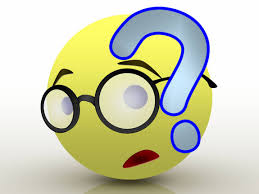# Paralyzing Progressions!

Algebra Level 2Find the sum of the values of $x$ and $y$ if $(x+y)$, $(2x+1)$, $(3x-1)$ are in $AP$ and $x$, $xy$ and $(3x+10y)$ are in $GP$.

×

Problem Loading...

Note Loading...

Set Loading...